# The Door Opener: Matrices and Gauss-Jordan Elimination

Imagine learning something back when you were a kid, like lets say, riding a bike. You’d know a certain set of steps needed to accomplish the task such as sitting on the bike or pedalling on the pedals. You’d also know how difficult or easy it is since that’s what you’ve been doing your whole life up to that point.

## What is a System of Equations?

A system of equations is exactly what its name implies: a set of one or more equations that contain a number of unknown variables. The goal of these kinds of problems is to look for all possible values of the unknown variables, such that it satisfies all the equations that were given.

## What is a Linear Transformation?

I’ll be talking a lot about Linear Transformations later on in the blog, but for now, here’s a small smidget so we have an idea of what’s going on.

## Gauss-Jordan Elimination

Now that we have those ideas out of the way, let’s dive into the process of how exactly Gauss-Jordan Elimination works. The easiest way to learn this, in my opinion, is by example, so here’s one for us to work with: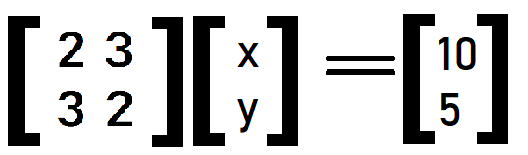We can represent this in terms of Ac=b (normally its Ax=b, but I’m already using x, so we’ll use c instead)

## What are EROs?

In total, there are three EROs, each represented by e or epsilon.

1. e1: Swapping two rows.
2. e2: Multiplying each number of a row by a non-zero number.
3. e3: Multiplying each number of a row by a non-zero number, then adding it to another row.
1. e2: r2 = (2)r2
2. e3: r2 = r2 + -(3)r1
3. e2: r1 = (½) r1
4. e2: r2 = -(⅕) r2
1. e3: r1 = r1 + (-3/2) r2

## Why this over solving it normally?

Before we get into technicalities such as optimization and the like, I’d personally like to say that I find this way of solving it to be more fun. With this, I actually understand what’s happening and the reasoning behind it. I also get to draw matrices, which for me is way more exciting than doing some algebraic substitutions and whatnot.

## Why Gauss-Jordan over other methods?

Gauss-Jordan is not the only strategy of Linear Algebra introduced to us in CS130. We were actually taught Gaussian Elimination right before this, and LU Decomposition right after. I won’t discuss the other two methods here since that would take too much time, but these methods also solve for the same type of problems that Gauss-Jordan Elimination solves for.

## Real World Examples

Since we are solving systems of equations, these methods have instant use in the real world because of how prevalent these types of problems are in our society. Various problems are solved using systems of equations, such as chemical balancing and traffic flow, most notably circuit problems that solve for voltage, current, and the like.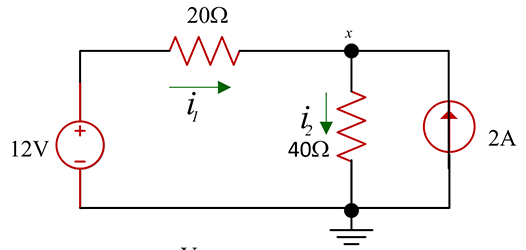an example of a circuit problem.

# Visualization: A Deeper Dive Into Linear Transformations

Earlier I said that we’d go deeper into Linear Transformations, so here we are. The reason I find this topic in particular to be so engaging is because its so visual. You can literally see the transformations happening in vectors when you graph it, and seeing it happen makes things way easier to understand.

1. F: v->w; where v and w are different vector spaces
2. F(u+v) = F(u) + F(v); where u and v are in the same vector space
3. F(au) = a * F(u); where a is a real number

## What is a vector space?

A vector space is, in its most simple terms, a set of objects, or vectors, that are enclosed within the normal rules of addition and multiplication. That is to say, vectors within a vector space are subject to the associative and commutative rules of addition, as well as the commutative rules of scalar multiplication.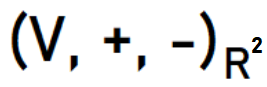V-set of real number vectors closed under addition and scalar multiplication.

## How exactly does that work?

Examples are always helpful, so here’s another one to help us out.

## Making things a bit more “matrix”-like

Linear transformations are not limited to the form of functions. In fact, all matrices, when acting as a multiplier to another matrix, are considered linear transformations as well! This is provided that if we had a linear transformation, f(v), where v is in the n dimensional vector space of all real numbers, then f(v) = Av, where A is the matrix representation of the function.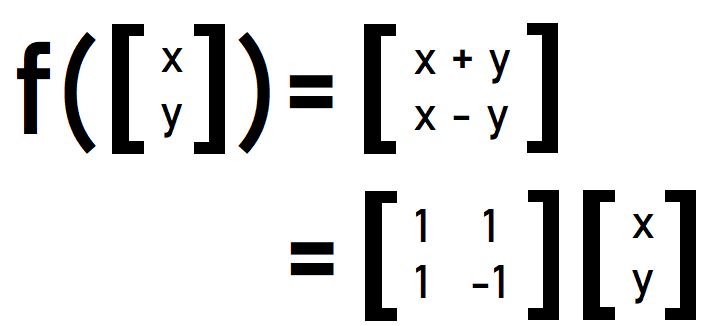f(v) = Av, where A is the matrix representation of f.

## Wait, what’s actually happening?

A key thing to note here is that when we are transforming a vector, we aren’t actually manipulating the vector itself. You can think of it more as manipulating the space in which the vector is based on, and what we’re solving for is what the vector would look like if we were to manipulate that space.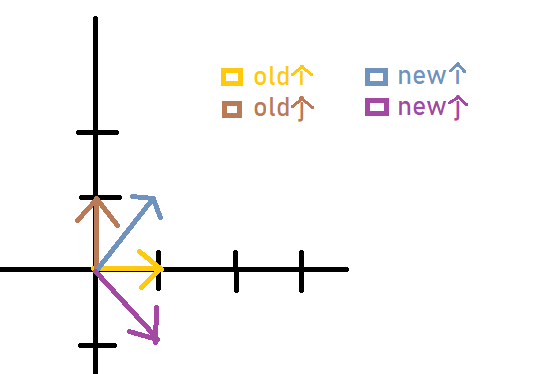i-hat is now at (1,1) from (1,0), while j-hat is now at (1,-1) from (0,1)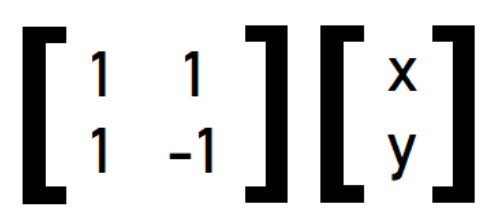(1,1)-first row, (1,-1)-second row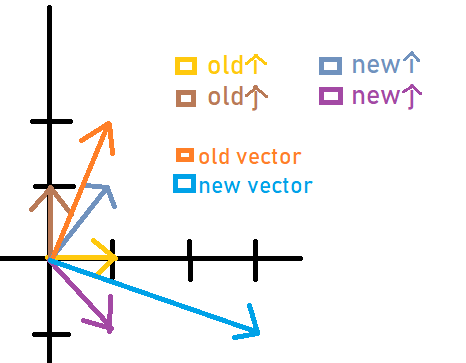fusion of everything I drew earlier.

## Application in 2D graphics

Before I continue with this, I’m not saying that linear transformations are limited to 2D graphics. We established earlier that this could work with any n dimensions of vector spaces as long as the rules are followed. I just want to use 2D since it is easier to draw.

## Scaling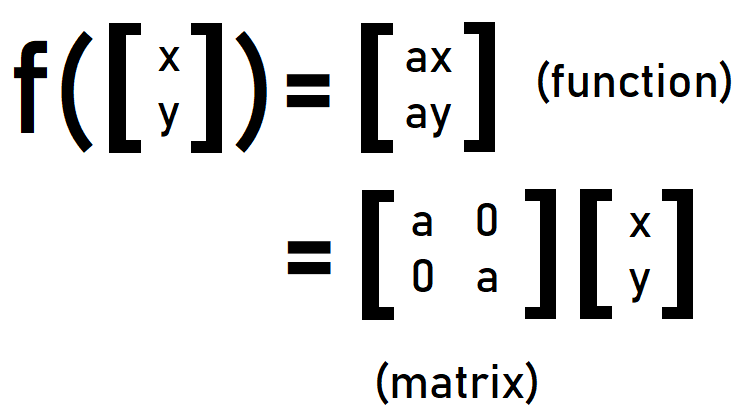a-scaling constant (ie. a=2: scale up by a factor of 2)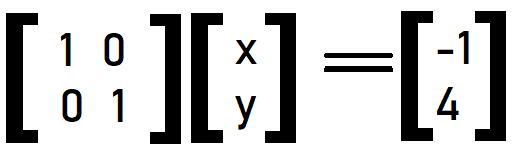Earlier example: [x y] = [-1 4], x=-1, y=4

# A Sudden Shift: Exploring Love with Differential Equations

Though I would have loved to talk more about the topics in the previous unit, I can confidently say that the same cannot be said for this next set of topics haha :D